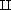Digital & Computing

### Become an OU studentData and processes in computing

Start this free course now. Just create an account and sign in. Enrol and complete the course for a free statement of participation or digital badge if available.

# 3.3 Mixing different forms of data: disjoint union of sets

At the supermarket checkout, some items need to be weighed (organic courgettes for example) and some do not. Let BarcodedItems be the set of items that do not need to be weighed, and WeighedItems be the set of items that must be weighed. When a weighed item is recorded at the till, we must record both the item type and the weight of the item that has been purchased. Earlier, we saw that such a purchase can be seen as an ordered pair, such as (“WALNUTS”, 335), that comes from the set WeighedItems × Int.

Suppose now that we want to form the set of all items that might appear in a transaction at a till. We might call this set TillItems. Specifying this set TillItems poses a complication, since there are two different types of element that might appear in it. An item from the set TillItems will come either from BarcodedItems or from WeighedItems × Int. We express this relationship by saying that TillItems is the disjoint union of BarcodedItems and WeighedItems × Int. We write this as:

You can read XY as “X or Y.”

TillItems = BarcodedItems(WeighedItems × Int).

In general, the disjoint union of sets X and Y , written XY , is the set consisting of all items that are either from X or from Y . The term “disjoint” reflects the fact that an item could not come both from BarcodedItems and from WeighedItems × Int. These sets contain different forms of data and have nothing in common. (We will only use disjoint union to combine sets containing different forms of data.)

As in Section 1, suppose that till1 is a variable representing a transaction in progress at till 1. The state of till1 will give the items recorded so far, in the order in which they were entered into the till, either by reading the barcode, or as a weighed item. So we can describe the state of till1 as a sequence of till items. The set of all possible states of till1 is SeqOfTillItems, where TillItems is BarcodedItems(WeighedItems × Int).

As noted earlier, we usually want to avoid mixing data of different forms in a collection such as a sequence. But if we need to do this, we can first use a disjoint union to combine the different forms of data into a single set. So, for example, if we needed to form a sequence whose members might be either characters or integers, then this sequence would come from a set SeqOfMix, where Mix is the disjoint union IntChar.

## Activity 12

Let TillItems = BarcodedItems(WeighedItems × Int), and suppose that BarcodedItems is represented as the set of integers between 10000 and 99999 and WeighedItems as the set of integers between 100 and 999. Which of the sequences given in (a)–(c) below is a member of the set SeqOfTillItems?

• (a) [1, −740, (22, 300)]

• (b) [11, ‘2’, ‘w’, 33000, −22]

• (c) [11023, 11023, (998, 12), 22375, (217, 147)]

### Discussion

Only the sequence in (c) is in SeqOfTillItems.

• (a) According to the statement, a barcoded item is represented by an integer with five digits. So 1 and 740 are not from the set BarcodedItems.

• (b) This sequence contains some characters, which are neither from the set BarcodedItems nor from WeighedItems × Int.

• (c) Each of the integers 11023 and 22375 lies between 10000 and 99999, and so comes from the set BarcodedItems. The first entry in each of the pairs (998, 12) and (217, 147) is an integer between 100 and 999, so comes from WeighedItems. Thus each of these ordered pairs comes from WeighedItems × Int. So each item in the sequence is either from BarcodedItems or from WeighedItems × Int, and so comes from TillItems.